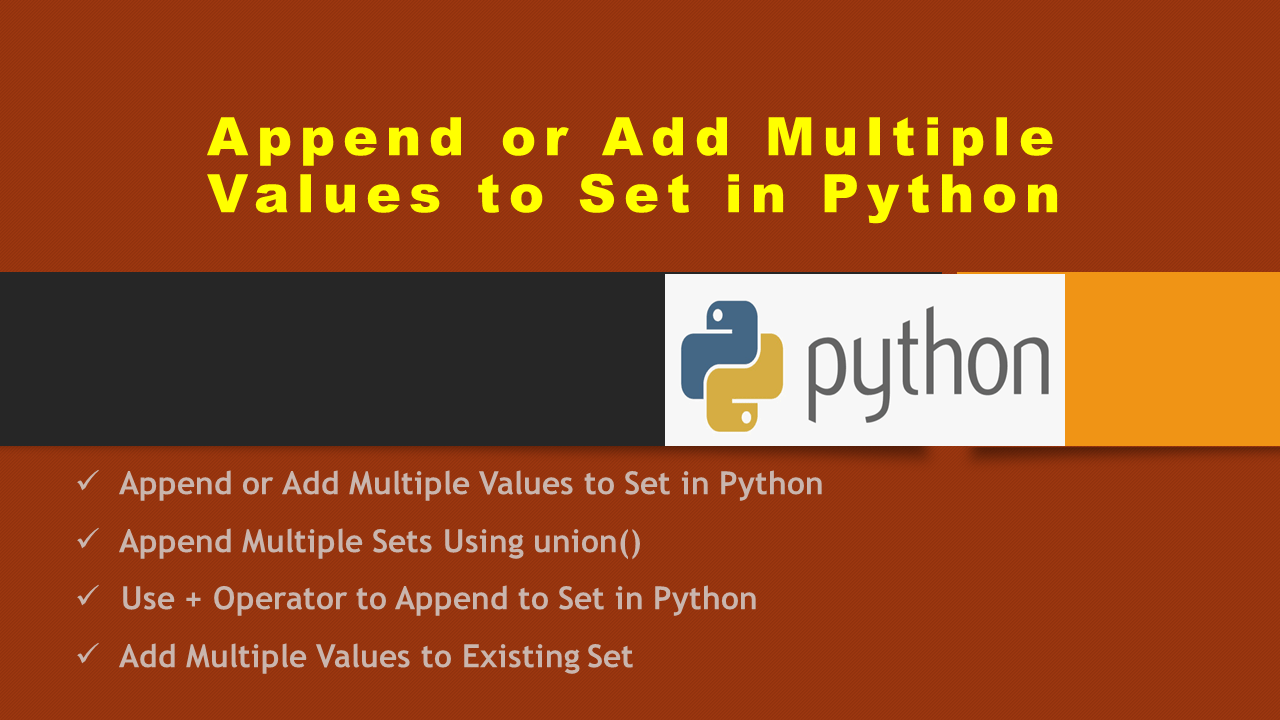# Append or Add Multiple Values to Set in Python

How to add or append multiple values to Set at a time in Python? In Python, there are several ways to append or add multiple values by using the + operator, set.union() function, and union (|) operator. Besides these, you can also use the update() method to add multiple sets to the existing set.

## 1. Quick Examples of Add Multiple Values to Set

Following are quick examples of appending or adding multiple values to the set.

``````
# Quick Examples

# Append using |
myset = myset1 | {10,20} | {30,40}

# Append using union()
myset = myset1.union({10,20},{30,40})

# Append using +
myset = set(list({30,40}) + list({30,40}))

# Append using update()
myset.update({30,40},{30,40})
``````

## 2. Append or Add Multiple Values to Set in Python

By using the `|` operator you can add or append multiple values to the set at a time in Python. Note that this operator works with only sets hence you need to have a set that you wanted to append and it returns results without duplicate values.

Let’s use the | operator to add two sets into a single set in Python. This would return a new set that contains all of the elements from `myset1` and `myset2`, without any duplicates.

``````
# Create sets
myset1 = {1,2,3,4,5,6}

# Append multiple sets operator |
myset = myset1 | {10,20}
print(myset)

# Output:
# {1, 2, 3, 4, 5, 6, 10, 20}
``````

Let’s also see another example with Strings. Here, I will append elements from multiple sets (3 sets) into a single set.

``````
# Create sets
set1 = {"one","two","three"}

# Append multiple sets using |
myset = set1 | {"four","five","six"} | {"nine"}
print(myset)

# Output:
# {'four', 'nine', 'one', 'three', 'six', 'two', 'five'}
``````

## 3. Append Multiple Sets Using union()

The set.union() is the same as the | operator, which will append values from multiple sets. Let’s use the same sets that were created above in the below example.

``````
# Append using union
myset = myset1.union({7,8,9})
print(myset)

# Output:
# {1, 2, 3, 4, 5, 6, 7, 8, 9}
``````

Similarly, you can append multiple sets with strings.

``````
# Append sets with string using union()
myset = set1.union({"four","five","six"},{"nine"})
print(myset)

# Output:
# {'four', 'nine', 'one', 'three', 'six', 'two', 'five'}
``````

## 4. Use + Operator to Append to Set in Python

The ‘+’ operator is used to append single or multiple sets at a time in Python. We can’t use sets with the + operator hence, we need to convert the set to a list, append lists and convert the result from the list to a set.

Let’s create two sets with integer values and append them into a single set. To perform the conversion, I will use list() to convert the set to a list and set() to convert the resulting list to a set.

``````
# Append  using + operator
myset = set(list(myset1) + list({10,20}))
print(myset)

# Output:
# {1, 2, 3, 4, 5, 6, 10, 20}
``````

Let’s see another example with strings.

``````
# Append  using +
myset = set(list(set1) + list({"four","five","six"}) + list({"nine"}))
print(myset)

# Output:
{'one', 'six', 'two', 'five', 'four', 'nine', 'three'}
``````

## 5. Add Multiple Values to Existing Set

All the above examples we have seen so far return a new set after appending multiple elements. To append multiple elements to the existing set use set.update() method.

``````
# Append elements to the existing set
myset1.update({10,20})
print(myset1)

set1.update({"four","five","six"})
print(set1)

# Output:
# {1, 2, 3, 4, 5, 6, 20, 10}
# {'four', 'one', 'three', 'six', 'two', 'five'}
``````

## 6. Conclusion

In this article, you have learned different ways to append or add multiple values to the set in Python by using the |, union(), update(), and + operators. Most of these return a new set after appending elements and to append to an existing set use the update() function. Make sure that you need to convert the set to a list before applying the ‘+’ operator. Both ‘|’ and union() functionality is the same.

Happy Learning!!

### Naveen

I am a Data Engineer with 20+ years of experience in transforming data into actionable insights. Over the years, I have honed my expertise in designing, implementing, and maintaining data pipelines with frameworks like Apache Spark, PySpark, Pandas, R, Hive and Machine Learning. My journey in the field of data engineering has been a continuous learning, innovation, and a strong commitment to data integrity. I have started this SparkByExamples.com to share my experiences with the data as I come across. You can learn more about me at LinkedIn